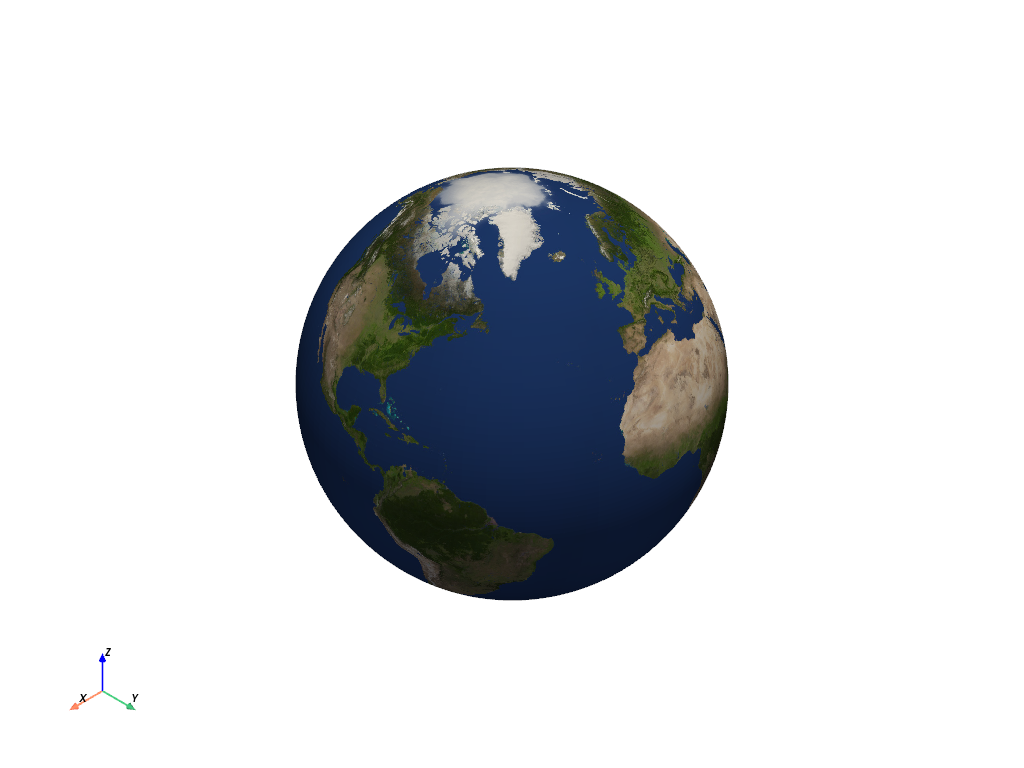# Applying Textures#

Plot a mesh with an image projected onto it as a texture.

```from matplotlib.cm import get_cmap
import numpy as np

import pyvista as pv
from pyvista import examples
```

Texture mapping is easily implemented using PyVista. Many of the geometric objects come preloaded with texture coordinates, so quickly creating a surface and displaying an image is simply:

```# load a sample texture

# create a surface to host this texture
surf = pv.Cylinder()

surf.plot(texture=tex)
```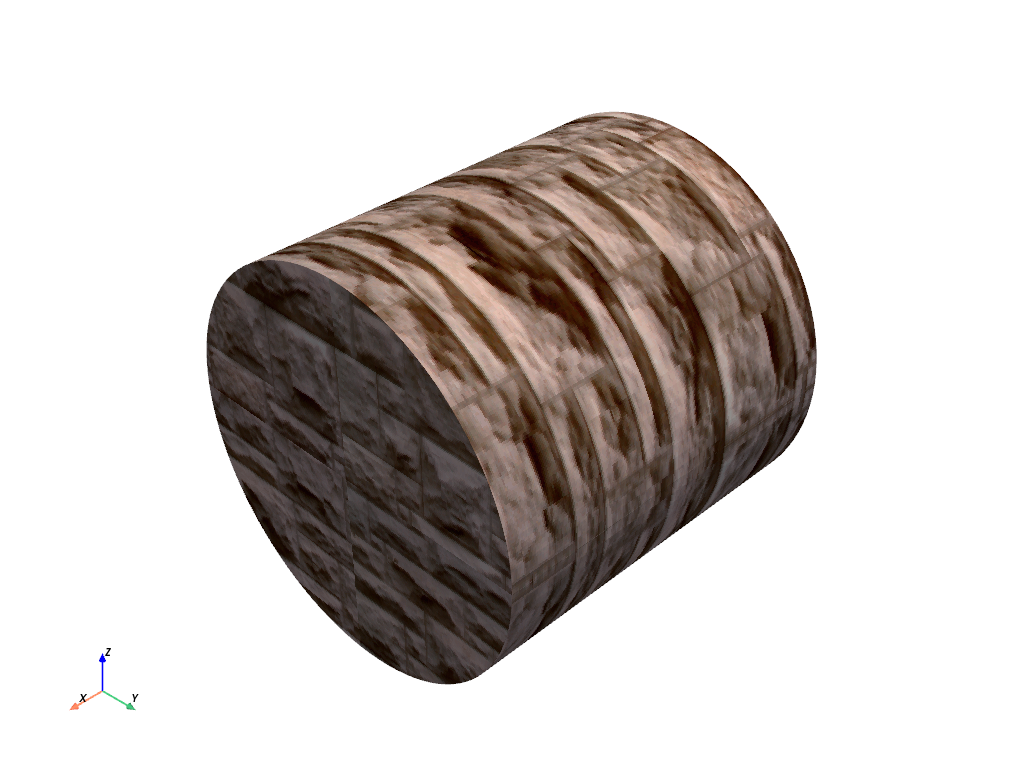But what if your dataset doesn’t have texture coordinates? Then you can harness the `pyvista.DataSetFilters.texture_map_to_plane()` filter to properly map an image to a dataset’s surface. For example, let’s map that same image of bricks to a curvey surface:

```# create a structured surface
x = np.arange(-10, 10, 0.25)
y = np.arange(-10, 10, 0.25)
x, y = np.meshgrid(x, y)
r = np.sqrt(x**2 + y**2)
z = np.sin(r)
curvsurf = pv.StructuredGrid(x, y, z)

# Map the curved surface to a plane - use best fitting plane
curvsurf.texture_map_to_plane(inplace=True)

curvsurf.plot(texture=tex)
```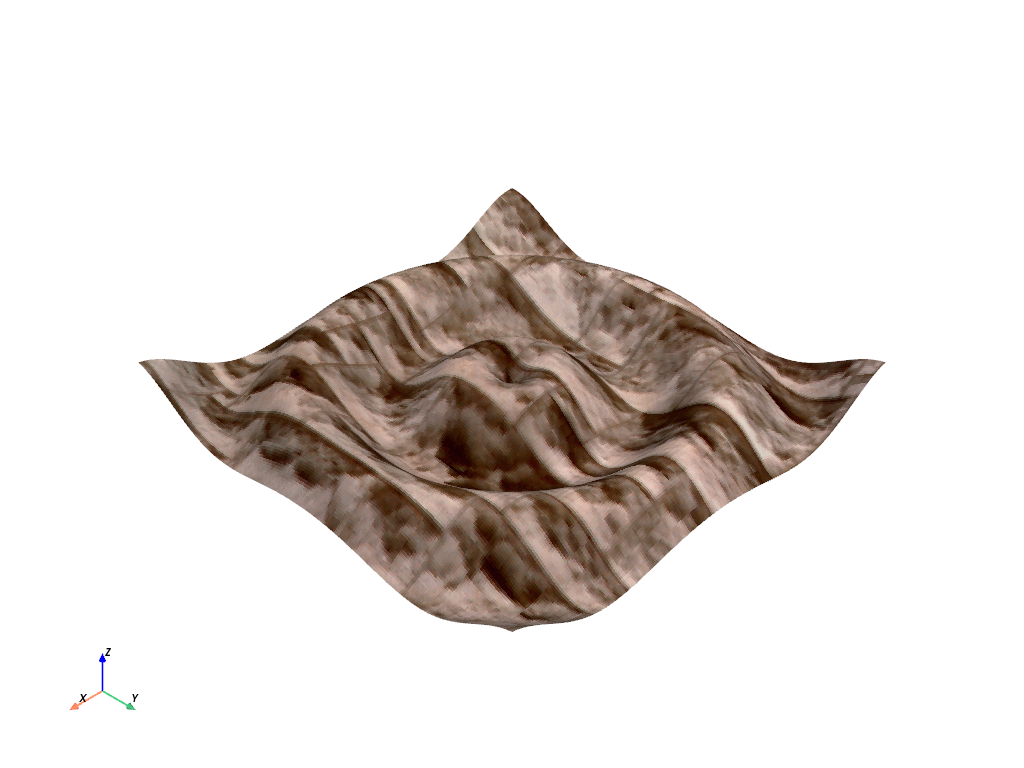Display scalar data along with a texture by ensuring the `interpolate_before_map` setting is `False` and specifying both the `texture` and `scalars` arguments.

```elevated = curvsurf.elevation()

elevated.plot(scalars='Elevation', cmap='terrain', texture=tex, interpolate_before_map=False)
```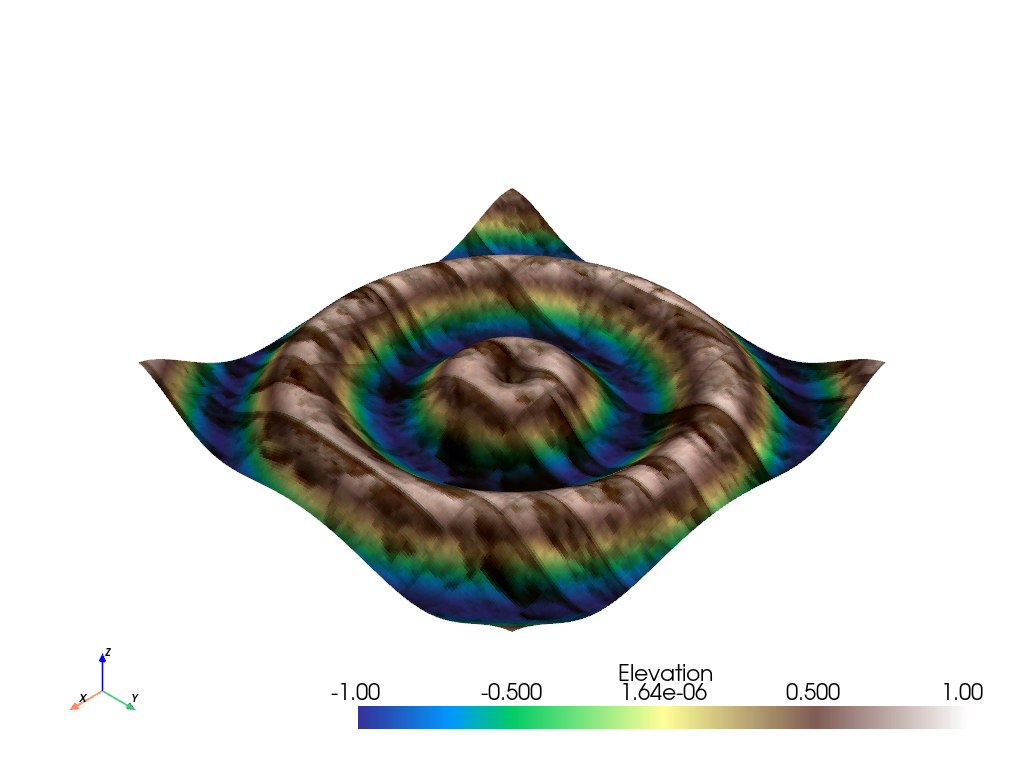Note that this process can be completed with any image texture.

```# use the puppy image
curvsurf.plot(texture=tex)
```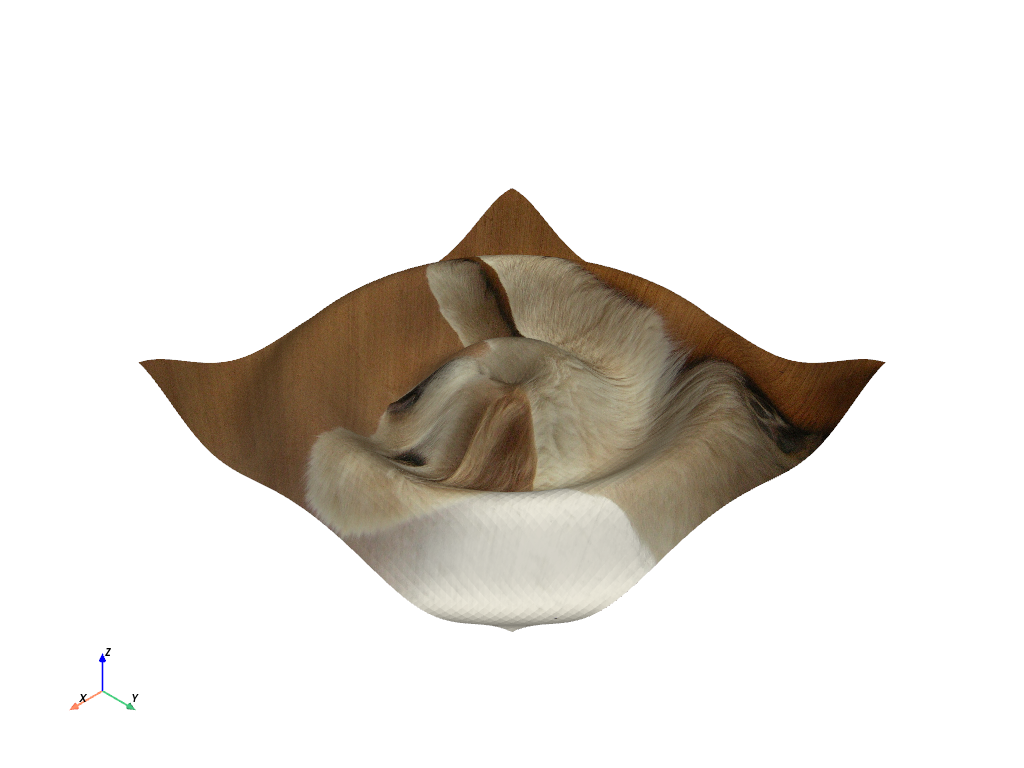## Textures from Files#

What about loading your own texture from an image? This is often most easily done using the `pyvista.read_texture()` function - simply pass an image file’s path, and this function with handle making a `vtkTexture` for you to use.

```image_file = examples.mapfile
curvsurf.plot(texture=tex)
```## NumPy Arrays as Textures#

Want to use a programmatically built image? `pyvista.UniformGrid` objects can be converted to textures using `pyvista.image_to_texture()` and 3D NumPy (X by Y by RGB) arrays can be converted to textures using `pyvista.numpy_to_texture()`.

```# create an image using numpy,
xx, yy = np.meshgrid(np.linspace(-200, 200, 20), np.linspace(-200, 200, 20))
A, b = 500, 100
zz = A * np.exp(-0.5 * ((xx / b) ** 2.0 + (yy / b) ** 2.0))

# Creating a custom RGB image
cmap = get_cmap("nipy_spectral")
norm = lambda x: (x - np.nanmin(x)) / (np.nanmax(x) - np.nanmin(x))
hue = norm(zz.ravel())
colors = (cmap(hue)[:, 0:3] * 255.0).astype(np.uint8)
image = colors.reshape((xx.shape, xx.shape, 3), order="F")

# Convert 3D numpy array to texture
tex = pv.numpy_to_texture(image)

# Render it
curvsurf.plot(texture=tex)
``````/home/runner/work/pyvista/pyvista/examples/02-plot/texture.py:96: MatplotlibDeprecationWarning: The get_cmap function was deprecated in Matplotlib 3.7 and will be removed two minor releases later. Use ``matplotlib.colormaps[name]`` or ``matplotlib.colormaps.get_cmap(obj)`` instead.
cmap = get_cmap("nipy_spectral")
```

## Create a GIF Movie with updating textures#

Generate a moving gif from an active plotter with updating textures.

```# Create a plotter object
plotter = pv.Plotter(notebook=False, off_screen=True)

# Open a gif
plotter.open_gif("texture.gif")

pts = curvsurf.points.copy()

# Update Z and write a frame for each updated position
nframe = 15
for phase in np.linspace(0, 2 * np.pi, nframe + 1)[:nframe]:
# create an image using numpy,
z = np.sin(r + phase)
pts[:, -1] = z.ravel()

# Creating a custom RGB image
zz = A * np.exp(-0.5 * ((xx / b) ** 2.0 + (yy / b) ** 2.0))
hue = norm(zz.ravel()) * 0.5 * (1.0 + np.sin(phase))
colors = (cmap(hue)[:, 0:3] * 255.0).astype(np.uint8)
image = colors.reshape((xx.shape, xx.shape, 3), order="F")

# Convert 3D numpy array to texture
tex = pv.numpy_to_texture(image)

plotter.update_coordinates(pts, render=False)

# must update normals when smooth shading is enabled
plotter.mesh.compute_normals(cell_normals=False, inplace=True)
plotter.write_frame()
plotter.clear()

# Closes and finalizes movie
plotter.close()
```## Textures with Transparency#

Textures can also specify per-pixel opacity values. The image must contain a 4th channel specifying the opacity value from 0 [transparent] to 255 [fully visible]. To enable this feature just pass the opacity array as the 4th channel of the image as a 3 dimensional matrix with shape [nrows, ncols, 4] `pyvista.numpy_to_texture()`.

```rgba = examples.download_rgba_texture()
rgba.n_components
```
```4
```
```# Render it
curvsurf.plot(texture=rgba, show_grid=True)
```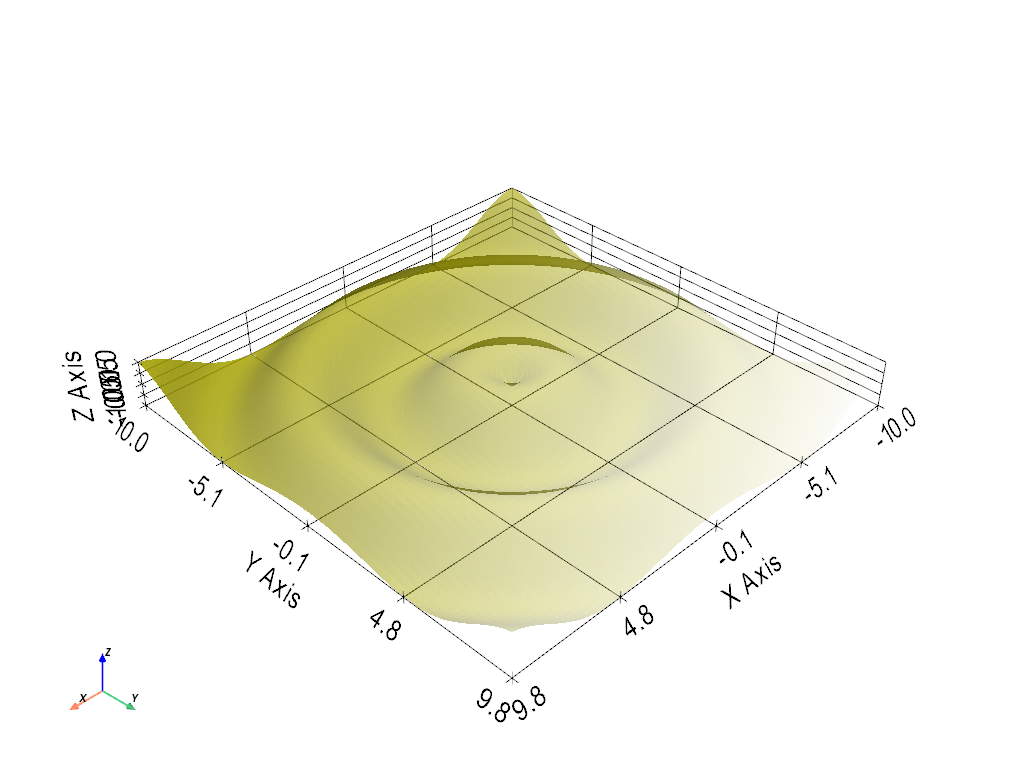## Repeating Textures#

What if you have a single texture that you’d like to repeat across a mesh? Simply define the texture coordinates for all nodes explicitly.

Here we create the texture coordinates to fill up the grid with several mappings of a single texture. In order to do this we must define texture coordinates outside of the typical `(0, 1)` range:

```axial_num_puppies = 4
xc = np.linspace(0, axial_num_puppies, curvsurf.dimensions)
yc = np.linspace(0, axial_num_puppies, curvsurf.dimensions)

xxc, yyc = np.meshgrid(xc, yc)
puppy_coords = np.c_[yyc.ravel(), xxc.ravel()]
```

By defining texture coordinates that range `(0, 4)` on each axis, we will produce 4 repetitions of the same texture on this mesh.

Then we must associate those texture coordinates with the mesh through the `pyvista.DataSet.active_t_coords` property.

```curvsurf.active_t_coords = puppy_coords
```

Now display all the puppies.

```# use the puppy image
curvsurf.plot(texture=tex, cpos="xy")
```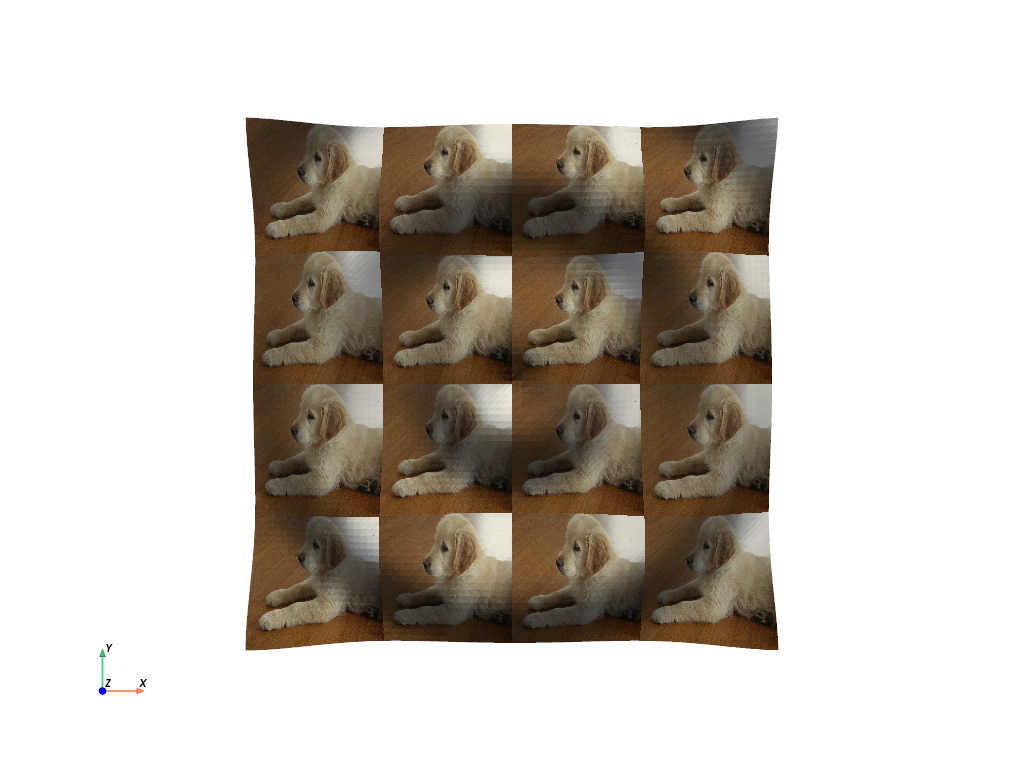## Spherical Texture Coordinates#

We have a built in convienance method for mapping textures to spherical coordinate systems much like the planar mapping demoed above.

```mesh = pv.Sphere()

mesh.texture_map_to_sphere(inplace=True)
mesh.plot(texture=tex)
```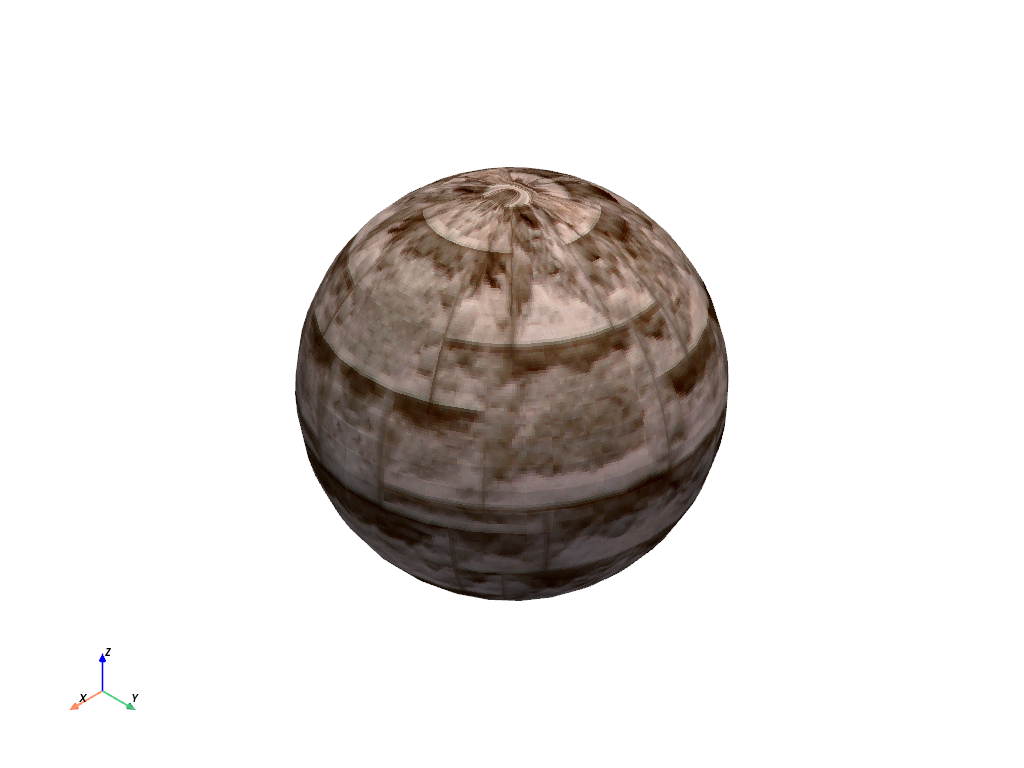The helper method above does not always produce the desired texture coordinates, so sometimes it must be done manually. Here is a great, user contributed example from this support issue

Manually create the texture coordinates for a globe map. First, we create the mesh that will be used as the globe. Note the start_theta for a slight overlappig

```sphere = pv.Sphere(
)

# Initialize the texture coordinates array
sphere.active_t_coords = np.zeros((sphere.points.shape, 2))

# Populate by manually calculating
for i in range(sphere.points.shape):
sphere.active_t_coords[i] = [
0.5 + np.arctan2(-sphere.points[i, 0], sphere.points[i, 1]) / (2 * np.pi),
0.5 + np.arcsin(sphere.points[i, 2]) / np.pi,
]

# And let's display it with a world map